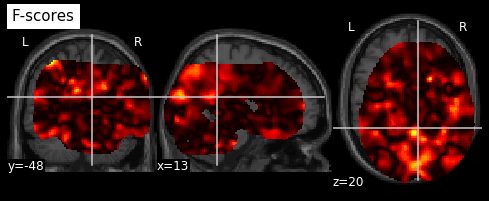# NiLearn Decoding, Masker - Shifted brain output on background image

Hi,
I am running a decoding analysis with NiLearn for the first time. The process seems fine but I am running into trouble with the image output of the decoding.

Here is what the output of my process looks like:This is a map of pvalues associated with the classification for a single subject. (I am performing a within-subject decoding).
All subject’s output images are similarly shifted.
I have used 2 differents masks: the MNI template as a mask, and an average of the subject’s masks from the univariate analyses (single-trial estimates).
Both give me skewed brains. The starting masks are fine, and when I view the initial masking procedure, there’s nothing off.

I suspect the inverse_transform procedure that flubs things? Any ideas?

Thank you.

Hi @LLK,

thank you very much for your post and welcome to neurostars, it’s great to have you here.

Is the output image in the same space as the image you want to overlay it on? Are the subject’s images all in the same (reference) space (e.g. MNI)? Could you possibly share the corresponding code snippets (`masker.fit/transform/inverse_transform`)? Based on the information you provided and the screenshot, I would assume something is off there…

Cheers, Peer

Hi Peer,
Thank you for the welcome.
Here is the code snippet for the masking step (i denotes subject index):

``````###redundant but keep it this way, in case you want to switch back to sub masks
for i in range(0,len(AllBetasS)):
for j in range (0, len(AllBetasS[i])):
#        report.open_in_browser()
``````

and then the inverse.transform part

``````for i in range(0,21):
sigP[i] = p_values[i] <0.05
p_values[i] = -np.log10(p_values[i])
p_values[i][p_values[i] > 10] = 10
sigF[i]=  f_values[i] * sigP[i]
f_score_img[i] = new_img_like(canImg, f_ma[i])
display= plot_stat_map(f_score_img[i],
title="F-scores", display_mode="ortho",
colorbar=False)
show()
display.close()
thisImg = f_score_img[i]
``````

All initial images used are in MNI space, as is the background image I use (‘canImg’). I do not have this problem when I run an SVC analysis on the same data and project coefficients onto the same image.
I have also tried not using an explicit background image and also checked the initial univariate masks in SPM. They all look fine…

Cheers!

Hi again,
I sorted it out. I am not sure what happened and don’t have time to dig too much right now, but streamlining the code fixed it up:

``````
for i in range(0,21):
sigP[i] = p_values[i] <0.05
p_values[i] = -np.log10(p_values[i])
p_values[i][p_values[i] > 10] = 10
sigF[i]=  f_values[i] * sigP[i]